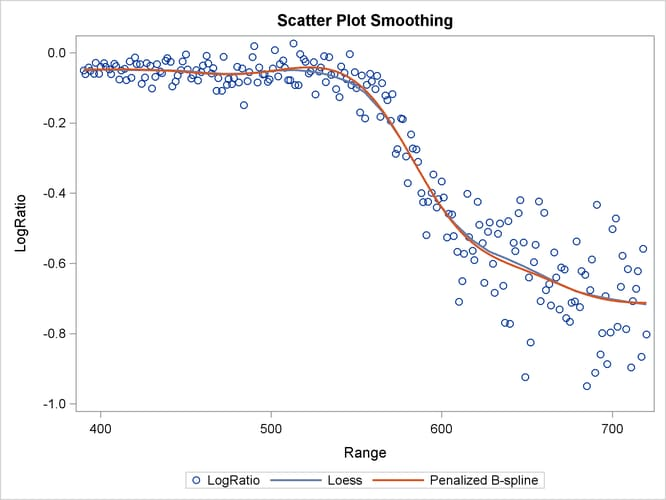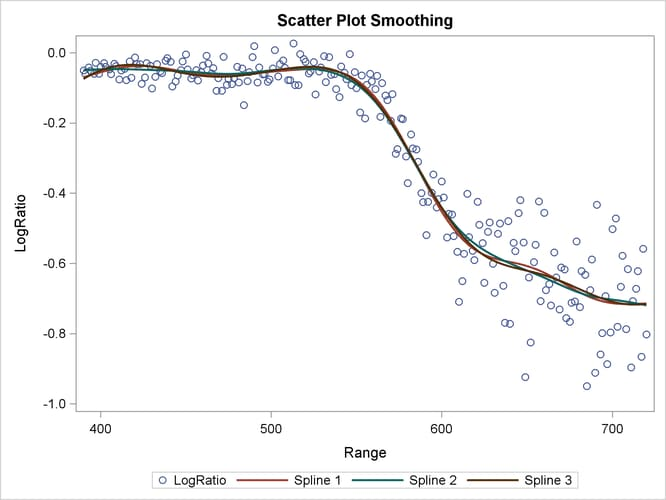# The GAMPL Procedure

### Example 7.1 Scatter Plot Smoothing

This example shows how you can use PROC GAMPL to perform scatter plot smoothing.

The example uses the LIDAR data set (Ruppert, Wand, and Carroll 2003). This data set is used in many books and journals to illustrate different smoothing techniques. Scientists use a technique known as LIDAR (light detection and ranging), which uses laser reflections to detect chemical compounds in the atmosphere. The following DATA step creates the data set `Lidar`:

```title 'Scatter Plot Smoothing';
data Lidar;
input Range LogRatio @@;
datalines;
390  -0.05035573    391  -0.06009706    393  -0.04190091    394   -0.0509847
396  -0.05991345    397  -0.02842392    399  -0.05958421    400  -0.03988881
402  -0.02939582    403  -0.03949445    405  -0.04764749    406     -0.06038
408  -0.03123034    409  -0.03816584    411  -0.07562269    412  -0.05001751
414   -0.0457295    415  -0.07766966    417  -0.02460641    418  -0.07133184

... more lines ...

702   -0.4716702    703   -0.7801088    705   -0.6668431    706   -0.5783479
708   -0.7874522    709   -0.6156956    711   -0.8967602    712   -0.7077379
714    -0.672567    715   -0.6218413    717   -0.8657611    718    -0.557754
720   -0.8026684
;
```

In this data set, `Range` records the distance that light travels before it is reflected back to the source. `LogRatio` is the logarithm of the ratio of light that is received from two laser sources. The objective is to use scatter plot smoothing to discover the nonlinear pattern in the data set. SAS provides different methods (for example local regression) for scatter plot smoothing. You can perform scatter plot smoothing by using the SGPLOT procedure, as shown in the following statements:

```proc sgplot data=Lidar;
scatter  x=Range y=LogRatio;
loess    x=Range y=LogRatio / nomarkers;
pbspline x=Range y=LogRatio / nomarkers;
run;
```

Output 7.1.1 shows the scatter plot of `Range` and `LogRatio` and the smoothing curves that are fitted by local regression and penalized B-splines smoothing techniques.

Output 7.1.1: Scatter Plot SmoothingBoth scatter plot smoothing techniques show a significant nonlinear structure between `Range` and `LogRatio` that cannot be easily modeled by ordinary polynomials. You can also use the GAMPL procedure to perform scatter plot smoothing on this data set, as in the following statements:

```proc gampl data=Lidar seed=12345;
model LogRatio = spline(Range/details);
output out=LidarOut pred=p;
run;
```

The “Specifications for Spline(Range)” table in Output 7.1.2 displays the specifications for constructing the spline term for `Range`. The maximum degrees of freedom is 10, which sets the upper limit of effective degrees of freedom for the spline term to be 9 after one degree of freedom is absorbed in the intercept. The order of the derivative in the penalty is 2, which means the unpenalized portion of the spline term involves polynomials with degrees up to 2.

Output 7.1.2: Spline Specification

 Scatter Plot Smoothing

The GAMPL Procedure

Specifications for Spline(Range)
Number of Variables 1
Rank of Penalty Approximation 10
Order of Derivative in the Penalty 2
Maximum Number of Knots 2000

The “Fit Statistics” table in Output 7.1.3 shows the summary statistics for the fitted model.

Output 7.1.3: Fit Statistics

Fit Statistics
Penalized Log Likelihood 251.44124
Roughness Penalty 0.00000426
Effective Degrees of Freedom 9.99988
Effective Degrees of Freedom for Error 211.00000
AIC (smaller is better) -482.88271
AICC (smaller is better) -481.83512
BIC (smaller is better) -448.90148
GCV (smaller is better) 0.00654

The “Estimates for Smoothing Components” table in Output 7.1.4 shows that the effective degrees of freedom for the spline term of `Range` is approximately 8 after the GCV criterion is optimized with respect to the smoothing parameter. The roughness penalty is small, suggesting that there is an important contribution from the penalized part of thin-plate regression splines beyond nonpenalized polynomials.

Output 7.1.4: Estimates for Smoothing Components

Estimates for Smoothing Components
Component Effective
DF
Smoothing
Parameter
Roughness
Penalty
Number of
Parameters
Rank of
Penalty
Matrix
Number of
Knots
Spline(Range) 7.99988 1.0000 4.263E-6 9 10 221

Because the optimal model is obtained by searching in a functional space that is constrained by the maximum degrees of freedom for a spline term, you might wonder whether PROC GAMPL produces a much different model if you increase the value. The following statements fit another model in which the maximum degrees of freedom is increased to 20:

```proc gampl data=Lidar seed=12345;
model LogRatio = spline(Range/maxdf=20);
output out=LidarOut2 pred=p2;
run;
```

Output 7.1.5 displays fit summary statistics for the second model. The model fit statistics from the second model are very close to the ones from the first model, indicating that the second model is not much different from the first model.

Output 7.1.5: Fit Statistics

 Scatter Plot Smoothing

The GAMPL Procedure

Fit Statistics
Penalized Log Likelihood 250.95136
Roughness Penalty 0.06254
Effective Degrees of Freedom 10.04384
Effective Degrees of Freedom for Error 209.03211
AIC (smaller is better) -481.87757
AICC (smaller is better) -480.82094
BIC (smaller is better) -447.74696
GCV (smaller is better) 0.00657

Output 7.1.6 shows that the effective degrees of freedom for the spline term of `Range` is slightly larger than 8, which is understandable because increasing the maximum degrees of freedom expands the functional space for model searching. Functions in the expanded space can provide a better fit to the data, but they are also penalized more because the roughness penalty value for the second model is much larger than the one for the first model. This suggests that functions in the expanded space do not help much, given the nonlinear relationship between `Range` and `LogRatio`.

Output 7.1.6: Estimates for Smoothing Components

Estimates for Smoothing Components
Component Effective
DF
Smoothing
Parameter
Roughness
Penalty
Number of
Parameters
Rank of
Penalty
Matrix
Number of
Knots
Spline(Range) 8.04384 23134.6 0.0625 19 20 221

The two fitted models are all based on thin-plate regression splines, in which polynomials that have degrees higher than 2 are penalized. You might wonder whether allowing higher-order polynomials yields a much different model. The following statements fit a third spline model by penalizing polynomials that have degrees higher than 3:

```proc gampl data=Lidar seed=12345;
model LogRatio = spline(Range/m=3);
output out=LidarOut3 pred=p3;
run;
```

The fit summary statistics shown in Output 7.1.7 are close to the ones from the previous two models, albeit slightly smaller.

Output 7.1.7: Fit Statistics

 Scatter Plot Smoothing

The GAMPL Procedure

Fit Statistics
Penalized Log Likelihood 249.79779
Roughness Penalty 9.440383E-9
Effective Degrees of Freedom 10.00000
Effective Degrees of Freedom for Error 211.00000
AIC (smaller is better) -479.59559
AICC (smaller is better) -478.54797
BIC (smaller is better) -445.61397
GCV (smaller is better) 0.00664

As shown in Output 7.1.8, the effective degrees of freedom for the spline term where polynomials with degrees less than 4 are allowed without penalization is 8. The roughness penalty is quite small compared to the previous two fits. This also suggests that there are important contributions from the penalized part of the thin-plate regression splines even after the nonpenalized polynomials are raised to order 3.

Output 7.1.8: Estimates for Smoothing Components

Estimates for Smoothing Components
Component Effective
DF
Smoothing
Parameter
Roughness
Penalty
Number of
Parameters
Rank of
Penalty
Matrix
Number of
Knots
Spline(Range) 8.00000 1.0000 9.44E-9 9 10 221

The following statements use the DATA step to merge the predictions from the three scatter plot smoothing fits by PROC GAMPL and use the SGPLOT procedure to visualize them:

```data LidarPred;
merge Lidar LidarOut LidarOut2 LidarOut3;
run;

proc sgplot data=LidarPred;
scatter x=Range y=LogRatio / markerattrs=GraphData1(size=7);
series  x=Range y=p        / lineattrs  =GraphData2(thickness=2)
legendlabel="Spline 1";
series  x=Range y=p2       / lineattrs  =GraphData3(thickness=2)
legendlabel="Spline 2";
series  x=Range y=p3       / lineattrs  =GraphData4(thickness=2)
legendlabel="Spline 3";
run;
```

Output 7.1.9 displays the scatter plot smoothing fits by PROC GAMPL under three different spline specifications.

Output 7.1.9: Scatter Plot Smoothing